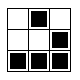Geometrize  1.0 An application for geometrizing images into geometric primitives
`#include <functional>`
`#include <memory>`
`#include <string>`
`#include <vector>`
`#include <QObject>`
`#include "geometrize/core.h"`
`#include "geometrize/bitmap/bitmap.h"`
`#include "geometrize/bitmap/rgba.h"`
`#include "geometrize/model.h"`
`#include "geometrize/shaperesult.h"`
`#include "geometrize/runner/imagerunneroptions.h"`
`#include "geometrize/shape/shape.h"`
`#include "preferences/imagetaskpreferences.h"`

Go to the source code of this file.

## Classes

The ImageTask class transforms a source image into a collection of shapes approximating the source image. More...

## Namespaces

geometrize

geometrize::script

chaiscript

## Functions

Q_DECLARE_METATYPE(std::vector< geometrize::ShapeResult >) Q_DECLARE_METATYPE(geometrize Q_DECLARE_METATYPE (geometrize::ShapeAcceptancePreconditionFunction)